# Chapter 6 Triangles NCERT Exemplar Solutions Exercise 6.1 Class 10 Maths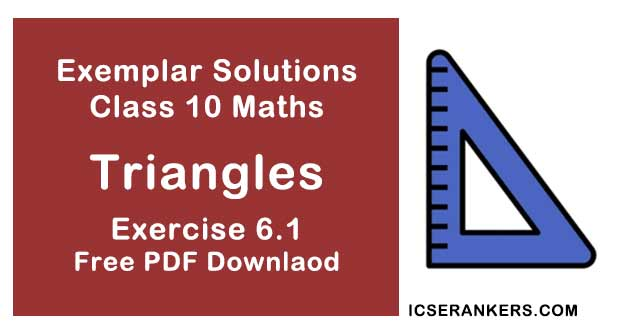Chapter Name NCERT Maths Exemplar Solutions for Chapter 6 Triangles Exercise 6.1 Book Name NCERT Exemplar for Class 10 Maths Other Exercises Exercise 6.2Exercise 6.3Exercise 6.4 Related Study NCERT Solutions for Class 10 Maths

### Exercise 6.1 Solutions

#### Multiple Choice Questions

Choose the correct answer from the given four options:

1. In figure, if ∠BAC = 90° and AD ⊥ BC . Then,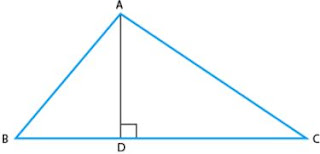If the lengths of the diagonals of rhombus are 16 cm and 12 cm. Then, the length of the sides of the rhombus is
(A) BD.CD = BC2
(B) AB.AC = BC2

Solution

We have,
∠D = ∠D = 90°  (∵ AD ⊥ BC)
∠DBA = ∠DAC  [each angle = 90°- ∠C]
From AAA similarity rule,
Therefore,

2. If the lengths of the diagonals of rhombus are 16 cm and 12 cm. Then, the length of the sides of the rhombus is
(A) 9 cm
(B) 10 cm
(C) 8 cm
(D) 20 cm

Solution

(B) 10 cm
We have,
A rhombus is a simple quadrilateral whose four sides are of same length and diagonals are perpendicular bisector of each other.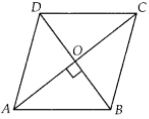Now,
AC = 16cm and
BD = 12 cm
∠AOB = 90°
AC and BD bisects each other
AO = 1/2 AC
BO = 1/2 BD
So,
AO = 8cm
BO = 6cm
In right angled ∆AOB,
By Pythagoras theorem,
We have,
AB2 = AO2 + OB2
⇒ AB2 = 82 + 62
= 64  36 = 100
⇒ AB = √100 = 10 cm
As the four sides of a rhombus are equal.
So, one side of rhombus = 10 cm.

3. If ∆ABC ∼ ∆EDF and ∆ABC is not similar to ∆DEF, then which of the following is not true ?

(A) BC.EF = AC.FD
(B) AB.EF = AC.DE
(C) BC.DE = AB.EF
(D) BC.DE = AB.FD

Solution

(C) BC.DE = AB.EF
If sides of one triangle are proportional to the side of the other triangle, and the corresponding angles are also equal, then the triangles are similar by SSS similarity.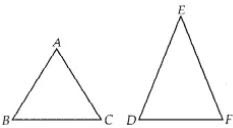So, ∆ABC ∼ ∆EDF
By similarity rule,
AB/ED = BC/DF = AC/EF
At first we take,
AB/ED = BC/DF
⇒ AB.DF = ED.BC
Hence, option (D) BC · DE = AB.FD is true
Now taking,
BC/DF = AC/EF , we get
⇒ BC.EF = AC.DF
Hence, option (A) BC.EF = AC.FD is true
Now if,
AB/ED = AC/EF, we get ,
⇒ AB.EF = ED.AC
Hence, option (B) AB.EF = AC.DE is true.

4. If in two triangles ABC and PQR, AB/QR = BC/PR = CA/PQ , then
(A) ∆PQR ~ ∆CAB
(B) ∆PQR ~ ∆ABC
(C) ∆CBA ~ ∆PQR
(D) ∆BCA ~∆PQR
Solution
(A) ∆PQR ~ ∆CAB
We have, from ∆ABC and ∆PQR,
AB/QR = BC/PR = CA/PQ
If sides of one triangle are proportional to the side of the other triangle, and their corresponding angles are also equal, then both the triangles are similar by SSS similarity.
So, we can say that,
∆PQR ~ ∆CAB

5. In fig. 6.3, two line segments AC and BD intersect each other at the point P such that PA = 6 cm, PB = 3 cm, PC = 2.5 cm, PD = 5 cm,  ∠APB = 50° and ∠CDP = 30° . Then, ∠PBA is equal to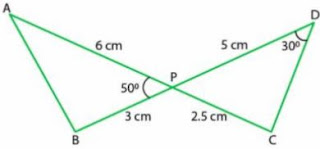(A) 50°
(B) 30°
(C) 60°
(D) 100°
Solution
(D) 100°
In ∆APB and ∆CPD,
∠APB = ∠CPD = 50°  (vertically opposite angles)
AP/PD = 6/5  ...(i)
And,
BP/CP = 3/2.5
⇒ BP/CP = 6/5 ...(ii)
From equations (i) and (ii),
AP/PD = BP/CP
Therefore,
∆APB ～∆DPC   [using SAS similarity rule]
∠A = ∠D = 30°  [Corresponding angles of similar triangles]
As,
Sum of angles of a triangle = 180°
From ∆APB,
∠A + ∠B + ∠APB = 180°
⇒ 30° + ∠B + 50° = 180°
⇒ ∠B = 180° – (50° + 30°)
⇒ ∠B = 180 – 80° = 100°
So, ∠PBA = 100°

6. If in two triangles DEF and PQR, ∠D = ∠Q and ∠R = ∠E, then which of the following is not true ?
(A) EF/PR = DF/PQ
(B) DE/PQ = EF/RP
(C) DE/QR = DF/PQ
(D) EF/RP = DE/QR
Solution
(B)
We have,In ∆DEF and ∆PQR,
∠D = ∠Q,
∠R = ∠E
∆DEF ～ ∆QRP
∠F = ∠P
DF/QP = ED/RQ = FE/PR

7. In triangles ABC and DEF, ∠B = ∠E, ∠F = ∠C and AB = 3DE. Then, the two triangles are
(A) Congruent but not similar
(B) Similar but not congruent
(C) Neither congruent nor similar
(D) Congruent as well as similar
Solution
(B)
In ∆ABC and ∆DEF,
∠B = ∠E,
∠F = ∠C and
AB = 3DE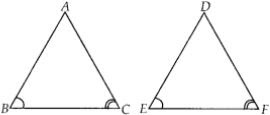We know that, if in two triangles corresponding two angles are same, then they are similar by
AA similarity criterion.
But,
AB ≠ DE
Therefore ∆ABC and ∆DEF are not congruent.

8. It is given that ∆ABC ～∆PQR , with BC/QR = 1/3, Then, ar(PRQ)/ar(BCA) is equal to
(A) 9
(B) 3
(C) 1/3
(D) 1/9
Solution
(A)
We have,
∆ABC ～ ∆PQR
BC/QR = 1/3
We know that, the ratio of the areas of two similar triangles is equal to square of the ratio of their corresponding sides.
Therefore,
ar(PRQ)/ar(BCA) = QR2/BC2
QR2 /BC2 = 32 /12 = 9

9. It is given that ∆ABC ～∆DFE,  ∠A = 30°, ∠C = 50°, AB = 5 cm, AC = 8cm and DF = 7.5 cm. Then, the following is true :
(A) DE = 12 cm, ∠F = 50°
(B) DE = 12 cm, ∠F = 100°
(C) EF = 12 cm, ∠D = 100°
(D) EF = 12 cm, ∠D = 30°
Solution
We have,
∆ABC ~ ∆DFE,
∠A = ∠D = 30°,
∠C = ∠E = 50°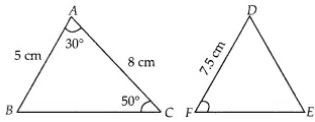∠B = ∠F
180° - (50° + 30°)
= 100°
Now,
AB/DF = AC/DE
⇒ 5/7.5 = 8/DE
⇒ DE = 12 cm

10. If in triangles ABC and DEF, AB/DE = BC/FD, then they will be similar when
(A) ∠B = ∠E
(B) ∠A = ∠D
(C) ∠B = ∠D
(D) ∠A = ∠F
Solution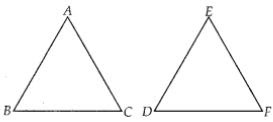Given, in ∆ABC and ∆EDF,
AB/DE = BC/FD
Therefore,
∆ABC ～ ∆EDF if, ∠B = ∠D  [By SAS similarity criterion]

11. If ∆ABC ～∆QRP, and BC = 15 cm, then PR is equal to
(A) 10 cm
(B) 12 cm
(C) 20/3 cm
(D) 8 cm
Solution
In given question,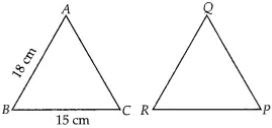We know that the ratio of area of two similar triangles is equal to the ratio of square of their
corresponding sides.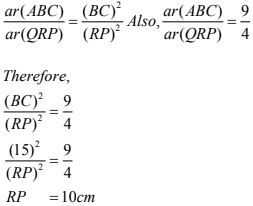12. If S is a point on side PQ of a ∆PQR such that PS = QS = RS, then
(A) PR . QR = RS2
(B) QS2 + RS2 = QR2
(C) PR2 + QR2 = PQ2
(D) PS2 + RS2 = PR2
Solution
In given question,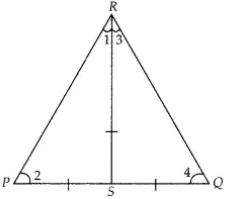In ∆PQR,
PS = QS = RS ...(i)
Now,
In ∆PSR,
PS = RS (By eqn (i))
∠1 = ∠2  ...(ii)
[Angles opposite to equal sides are equal]
Also, in ∆RSQ,
RS = SQ
∠3 = ∠4  ...(iii)
[angles opposite to equal sides are equal]
We know that, in ∆PQR, sum of angles = 180°
∠P + ∠Q + ∠P = 180°
⇒ ∠2 + ∠4 + ∠1 + ∠3 = 180°
⇒ ∠1 + ∠3 + ∠1 + ∠3 = 180°
⇒ 2(∠1 + ∠3) = 180°
⇒ (∠1 + ∠3) = 90°
So, ∠R = 90°
In ∆PQR, by Pythagoras theorem,
PR2 + QR2 = PQ2